Analysis WebNotes
arrow_back arrow_forward

# Class Contents

## Properties of Sequentially Compact Sets (continued)

### Applications to differentiable functions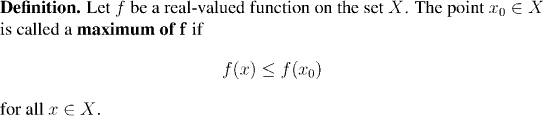We have seen that continuous functions defined on sequentially compact metric spaces always have maxima and minima. Of course there was no assumption that the functions in question were differentiable, and this, in itself is a remarkable fact: that every continuous function on a sequentially compact set has a maximum or a minimum, irrespective of whether or not it is differentiable.

Let us see what we can say about a differentiable function at a maximum or minimum: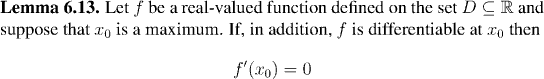The picture shows the key idea of the lemma's proof, that the slope of secant lines to the right of the maximum is negative and to the left of the maximum is negative. Since these slopes must converge to a common value, the limit must be zero.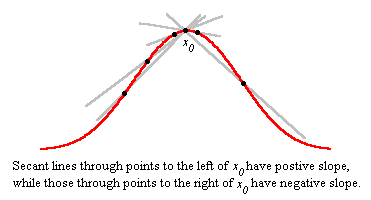Question: So, do Lemma 6.12 and Proposition 6.11 mean that it a function is differentiable at every point of [a,b] then it has to have a point where it has zero derivative?

Question: Can you explain why this is?

The next result shows what we can accomplish in this direction: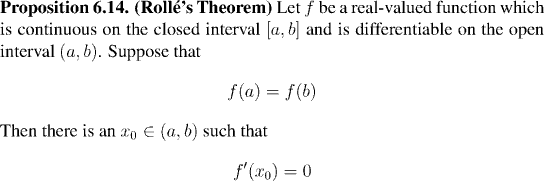Key ideas : domain of definition | continuous | differentiableThe idea of the proof.The proof itself.

Question: Can you see why the theorem is called the Mean Value theorem?By exactly the same arguments, we can prove: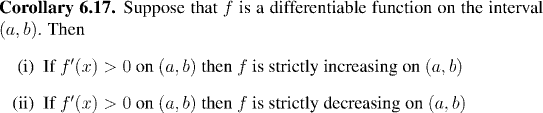We can bring together the results of several of our recent theorems in the following useful result. In fact this theorem is only a special case of another, bigger, theorem of the same name which we will (hopefully) get to when we study derivatives of functions of several variables.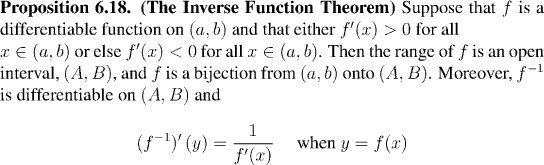Remark:

The endpoints of the intervals, a, b, A, B may very well be extended real numbers. In other words, unbounded open intervals are perfectly possible for one or other of (a,b) and (A,B).

As an application of this theorem, we shall prove that the same type of formula holds for differentiating x^q for q rational as we have seen holds for x^n when n is an integer.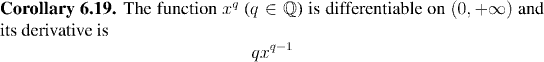Remark:

Note that we still do not know that the general power function xa for real a is differentiable and that its derivative is given by the formula from calculus: ax(a-1). We will have to wait until after we can prove that exponential functions are differentiable before we can do this, which will be in the next chapter.